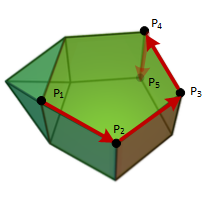# Simplex On Line Calculator. The on-line Simplex method Aplicattion.

### Basic concepts and principles

The application Simplex On Line Calculator is useful to solve linear programming problems as explained at Mathstools theory sections. It applies two-phase or simplex algorithm when required.

Do not enter slack or artificials variables, Simplex On Line Calculator does it for you.

Also, there is an Android version for Android devices on this link

Simplex On Line Calculator allows user to watch in detail step by step simplex execution and each one phase of two-phase method.### Extended TheoryThe simplex algorithm performs iterations between the extreme points set of feasible region, checking for each one if Optimalit criterion holds.
To use it properly, just rewrite your problem in standard form as explained at section Linear Programming .

The program window opens with a default problem, which has a finite optimal solution.
Application consists of the following menu:

1)Restart The screen back in the default problem.

2)Dualize Transforms the problem in its dual.

3)Add Column Add a column to constraints matrix (and hence to costs vector).

4)Add Row Add a row to constraints matrix (and therefore to Vector Constraints), ie anand dimension to problem.

5)Delete Column Deletes a column in the matrix (and therefore costs Vector).

6)Delete Row Deletes a row of the matrix (and therefore Restrictions Vector).

7)Execute Executes simplex algorithm and obtains the final solution.

8)Step-By Step Execute Executes simplex or two phase method allowing look each step and phase of the simplex algorithm.

## Was useful? want add anything?

Post here

### cassamo:

2012-12-06 08:14:27
crude refinery uses three (1,2 and 3) to produce four products (gasoline, kerosene, diesel and residual) Cost of raw materials and selling prices of the products are shown in the diagram.:

crude1($110/bbl) gasolina($360/bbl)
crude2($75/bbl) black box querosene($240/bbl)
crude3($90/bbl) gasoleo($210/bbl)
residuo($100/bbl) The following table shows the expected performance of each of the crude oil processed in terms of the desired products.  Product Yield by % of volume Desired maximum production bbl/day Crude1 Crude2 Crude3 Gasoline 80 60 44 24000 Kerosene 5 15 10 2000 Diesel 10 20 36 6000 Residue 5 5 10 Costs of processing ($/bbl) 5 5 10

a) Formulate the problem

b) terminate the simplex method and determine the optimal production plan for the refinery

Post here